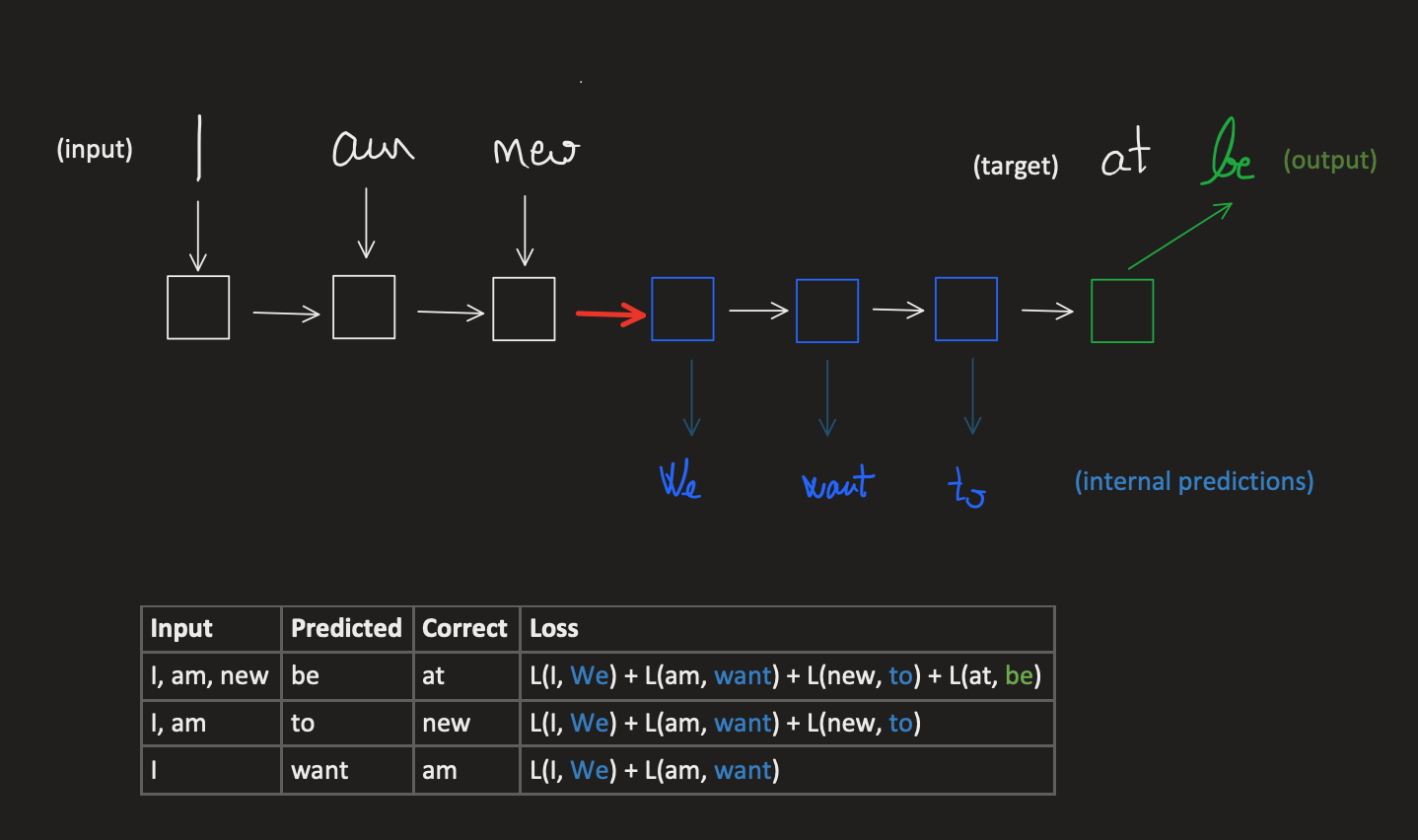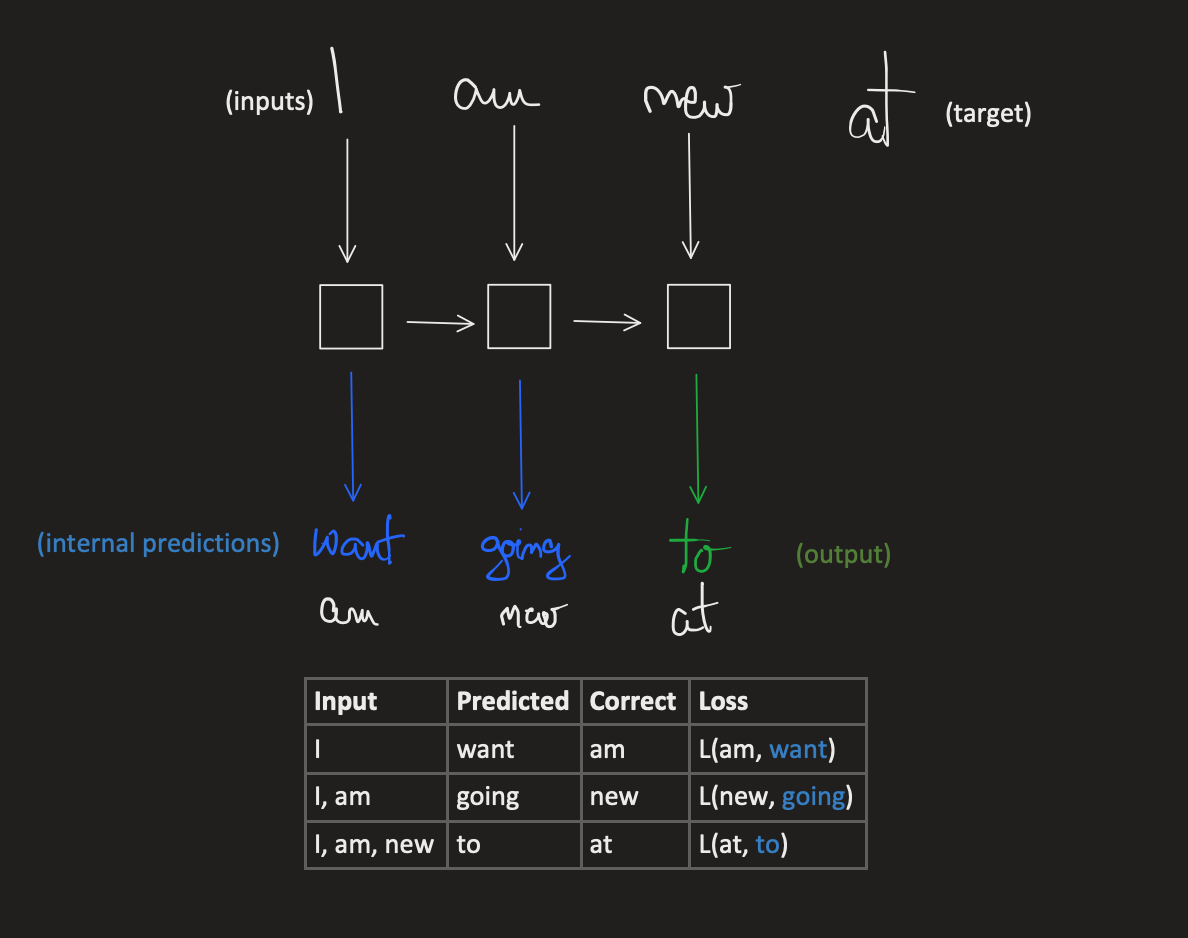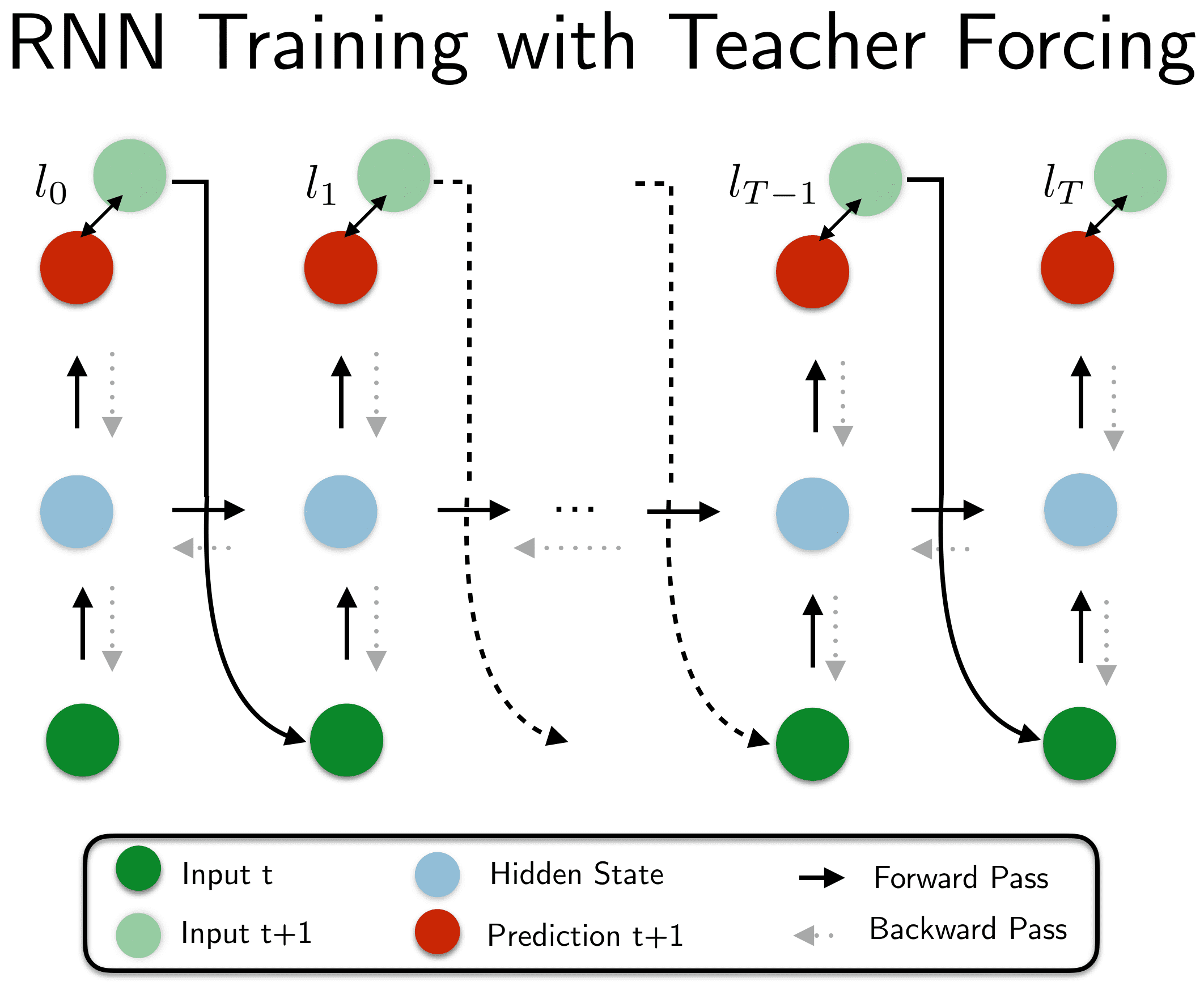# Training an RNN with teacher forcing.

20200608182759

Teacher forcing is a (really simple) way of #training an #rnn.

RNNs have a variable length input and this is by design, since this is why they are mainly used (to convert a sequence - like text - into a single encoding - #embedding).

## The problem

Suppose you have the input: `(w0, w1, w2, ..., wn)`

What you normally would do for training, maybe in a naive way, is to #autoregress, which means sending all the tokens of the input into the RNN and compute the loss as the result you get at the very end, then backpropagate through time, for all the steps with their relative partial-losses.

If you are in a #seq2seq model (say in #language_models or #machine_translation), where you want to predict a sequence, derived from the input sequence, doing the above ends you up with the following (logically unrolled) algorithm:

• Forward pass:
• passing `w0` into the RNN model and getting a prediction `p0`.
• this makes the RNN pass to a new state `s0` to reflect the output you just sent
• (internally) compute the partial-loss `l0`: between `p0` and the expected answer `e0`

• send in the next token `w1` into the RNN model and getting a prediction `p1`
• (internally) compute the partial-loss `l1`: between `p1` and the expected answer `e1`

• .… (repeat n times for all `w_i`)
• after all these steps, you now have the output prediction `(p0, p1, p2, ..n, pn)`, which you want to train so as to be as close as possible to the target (expected) sequence `(t0, t1, t2, ..., tn)`

• Backwards pass:
• backpropagate through time, using each `l_i` as the loss value for each timestep you’re at

So you have:

• `(w0, w1, w2, ..., wn)` - inputs
• `(p0, p1, p2, ..., pn)` - outputs
• `(t0, t1, t2, ..., tn)` - targets
• `(l0, l1, l2, ..., ln)` - partial-loss values

In this formulation, notice that `p1` is only as good as `p0` was, because `p1` used the RNN that yielded `p0`. Since usually, `p0` is way off (especially at the beginning of training) then `p1`, `p2` and the following have a increasingly smaller chance to land on the correct outputs (because they depend on the previous erroneous states).

## Teacher forcing

To overcome this, you actually need not continue on step `i` from the intermediary state `i-1` (that yields `p_i`) but from a state that received all the correct inputs, up to the current one. In other words, we need to make `p_i` dependent on the sequence`(t0, t1, t2, ..., t(i-1))` not on `(p0, p1, p2, ..., p(i-1))`.

Let’s take an example. Suppose we want to predict the next word in the sequence `(I, am, new)` which is `at`.What would normally happen is that you’d pass all the words into the RNN `I`, `am`, `new` and let the RNN build up an internal state representing this sequence, into an internal state (the red arrow above) and and then unroll this hidden state for 3 steps to get the final output.

Internally, at each unrolling step you actually get some intermediary predictions (in blue) and they depend on what the RNN unrolled previously in step `(t-1)`. This means that since `We` was a wrong prediction, `want` may be sensible as a next word, given `We` as a prior, but for the full sequence, it builds up on the previous error. By the time, we get to the output, we have accumulated all the errors of:

• predicting `be`, which depended on the error of predicting previously both `We` and `want` and `to`
• predicting `to` which depended on the error of predicting previously both `We` and `want`
• predicting `want` which depended on the error of predicting `We`

To correct these issues, the backpropagtion step has to compute the loss values as cummulative sums of all the loss values of previous predictions (#”backpropagation through time” #bptt).

Teacher forcing solves this by sending in all the correct predictions as priors, and only deal of forcing (correcting) a single step of the output. It’s as if, we are sending cummulative sequences as inputs, and expect the next token and only backpropagate for that loss value, as you can see in the image bellow.So, we compute the intermediary predictions, we act on their errors (through backpropagation) but only once, since from that point forward we’ll make next tokens depend on the correct previous tokens.

This speeds up training because now we have a linear training schedule, one word at a time.Credits for this image